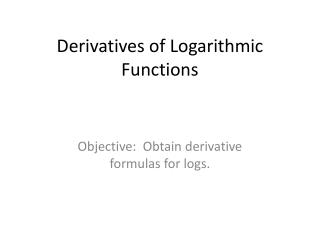Download PresentationDerivatives of Logarithmic Functions# Derivatives of Logarithmic Functions - PowerPoint PPT Presentation

Download Presentation##### Derivatives of Logarithmic Functions

Download Policy: Content on the Website is provided to you AS IS for your information and personal use and may not be sold / licensed / shared on other websites without getting consent from its author. While downloading, if for some reason you are not able to download a presentation, the publisher may have deleted the file from their server.

- - - - - - - - - - - - - - - - - - - - - - - - - - - E N D - - - - - - - - - - - - - - - - - - - - - - - - - - -
##### Presentation Transcript

1. Derivatives of Logarithmic Functions Objective: Obtain derivative formulas for logs.

2. Review Laws of Logs • Algebraic Properties of Logarithms • Product Property • Quotient Property • Power Property • Change of base

3. Definitions to Remember

4. Example 1 • Find dy/dx for each of the following:

5. Example 2 (Try) • Find

6. Example 3 (try) • Find

7. Example 4 • Find

8. Example 4 • Find • We will use our rules of logs to make this a much easier problem.

9. Example 4 • Now, we solve.

10. Logarithmic Differentiation • This is another method that makes finding the derivative of complicated problems much easier. • Find the derivative of

11. Logarithmic Differentiation • Find the derivative of • First, take the natural log of both sides and treat it like example 3.

12. Logarithmic Differentiation • Find the derivative of • First, take the natural log of both sides and treat it like example 3.

13. Logarithmic Differentiation • Find the derivative of

14. Homework • Pages 247-248 • 1-33 odd